# MATH 251: Calculus 3, SET8

## 13: Vectors Functions

### 13.4: Motion in Space; Velocity and Acceleration

These problems are done with the CAS. See Hand Solutions for details.

#### 1. [878/4]

Plane curve with velocity and acceleration vectors
syms t positive % wlog
r(t) = [t^2 1/t^2], v(t) = diff(r(t),t), a(t) = diff(v(t), t)
r(t) =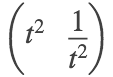v(t) =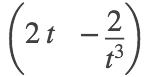a(t) =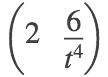r1 = r(1), v1 = v(1), a1 = a(1)
r1 =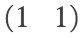v1 =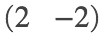a1 =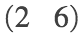speed = norm(v(t))
speed =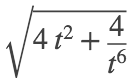%
figure
fplot(t^2, 1/t^2, [-0.5 2.5], 'LineWidth', 2); grid on; hold on
plot(1, 1, 'go', 'MarkerFaceColor', 'g', 'MarkerSize', 10)
quiver(1,1, 2,-2, 'r', 'LineWidth', 2, 'AutoScale', 'off', 'MaxHeadSize', 0.5) % velocity vector
quiver(1,1, 2,6, 'm', 'LineWidth', 2, 'AutoScale', 'off', 'MaxHeadSize', 0.2) % acceleration vector
axis equal; axis([-2 6 -2 8])
xlabel('x'); ylabel('y')
title('SET8, 878/4')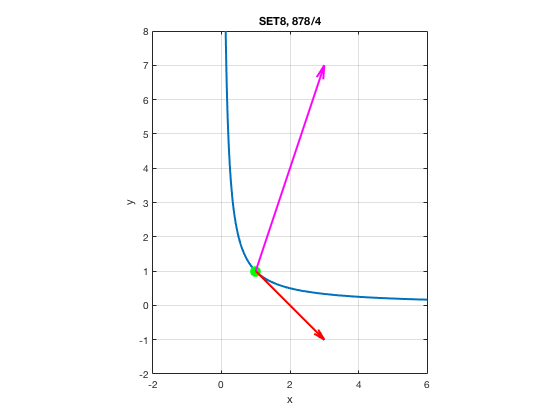#### 2. [878/8]

Space curve with velocity and acceleration vectors
syms t
r(t) = [t 2*cos(t) sin(t)], v(t) = diff(r(t),t), a(t) = diff(v(t), t)
r(t) =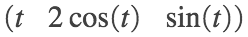v(t) =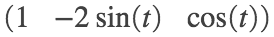a(t) =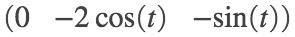r0 = r(0), v0 = v(0), a0 = a(0)
r0 =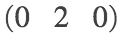v0 =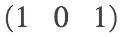a0 =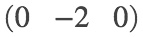speed = norm(v(t))
speed =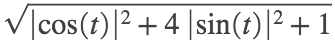%
figure
fplot3(t, 2*cos(t), sin(t), [-2*pi 2*pi], 'LineWidth', 2); grid on; hold on
plot3(0, 2, 0, 'go', 'MarkerFaceColor', 'g', 'MarkerSize', 10)
quiver3(0,2,0, 1,0,1, 'r', 'LineWidth', 2, 'AutoScale', 'off', 'MaxHeadSize', 0.8) % velocity vector
quiver3(0,2,0, 0,-2,0, 'm', 'LineWidth', 2, 'AutoScale', 'off', 'MaxHeadSize', 0.8) % acceleration vector
axis equal; axis([-2 6 -2 2 -1 1])
xlabel('x'); ylabel('y'); zlabel('z')
title('SET8, 878/8')
view(-26,26)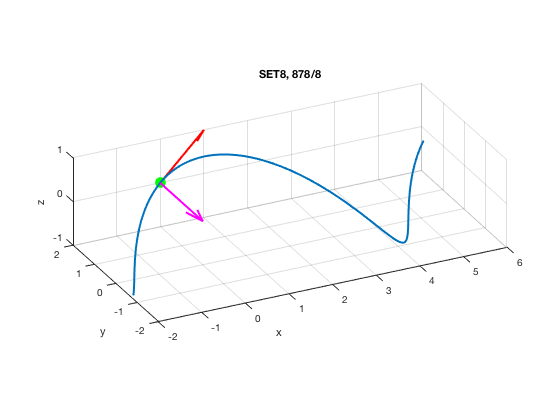#### 3. [878/12]

Given the position function, we compute velocity, acceleration, and speed once again.
syms t positive
r = [t^2 2*t log(t)]
r =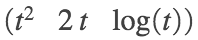v = diff(r,t), a = diff(v, t)
v =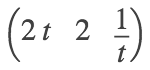a =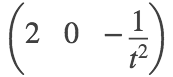speed = simplify(norm(v))
speed =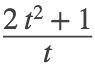#### 4. [878/16]

Given acceleration plus initial velocity and position, we obtain the position function.
See the Hand Solutions for how to do this step by step. (Or look at 861/42 in Section 13.2.)
Here we dispatch the problem in fully automatically by solving a system of differential equations with initial conditions, as in Math 308.
syms x(t) y(t) z(t)
Dx = diff(x,t), Dy = diff(y,t), Dz = diff(z,t)
Dx(t) =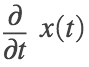Dy(t) =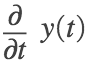Dz(t) =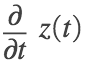deqs = [diff(x,t,2) == sin(t), ... % components of acceleration
diff(y,t,2) == 2*cos(t), ...
diff(z,t,2) == 6*t]
deqs(t) =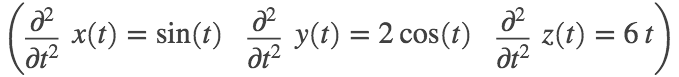conds = [Dx(0)==0, Dy(0)==0, Dz(0)==-1,... % initial velocity components
x(0)==0, y(0)==1, z(0)==-4] % initial position components
conds =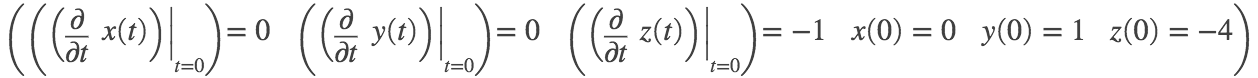soln = dsolve(deqs, conds) % Solve the initial value problem.
soln = struct with fields:
y: [1×1 sym] x: [1×1 sym] z: [1×1 sym]
x(t) = soln.x, y(t) = soln.y, z(t) = soln.z % Assign position components.
x(t) =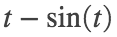y(t) =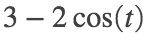z(t) =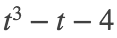r(t)= [x(t) y(t) z(t)] % Aggregate to obtain position vector function.
r(t) =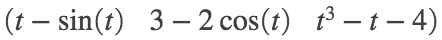#### 5. [878/18]

Same drill as 878/16 along with a graph of the position function, a space curve.
syms x(t) y(t) z(t)
Dx = diff(x,t), Dy = diff(y,t), Dz = diff(z,t)
Dx(t) =Dy(t) =Dz(t) =deqs = [diff(x,t,2) == t, ... % components of acceleration
diff(y,t,2) == exp(t), ...
diff(z,t,2) == exp(-t)]
deqs(t) =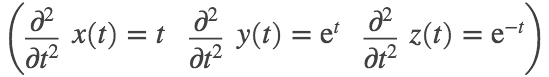conds = [Dx(0)==0, Dy(0)==0, Dz(0)==1, ... % initial velocity components
x(0)==0, y(0)==1, z(0)==1] % initial position components
conds =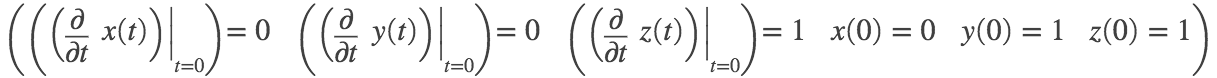soln = dsolve(deqs, conds) % Solve the initial value problem.
soln = struct with fields:
y: [1×1 sym] x: [1×1 sym] z: [1×1 sym]
x(t) = soln.x, y(t) = soln.y, z(t) = soln.z % Assign position components.
x(t) =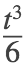y(t) =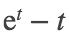z(t) =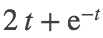r(t)= [x(t) y(t) z(t)] % Aggregate to obtain position vector function.
r(t) =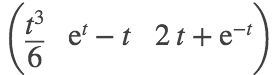%
figure
fplot3(t^3/6, exp(t)-t, 2*t+exp(-t), [-3.5 3.5], 'LineWidth', 2)
axis equal; % axis([-3 3 1 9 1 7])
xlabel('x'); ylabel('y'); zlabel('z')
title('SET8, 878/18')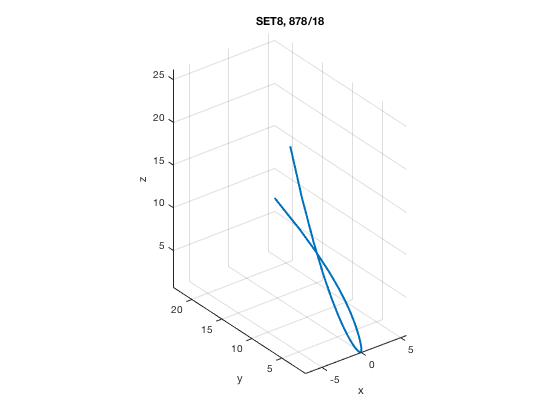% view(-26,26)

#### 6. [878/26]

A formula on page 873 relates distance with initial velocity and angle of elevation.
syms d g v0 alpha
assume(0 < alpha < pi/2)
eq1 = d == v0^2 * sin(2*alpha) / g
eq1 =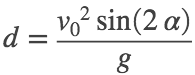eq2 = subs(eq1, [d g v0], [3000 98/10 400])
eq2 =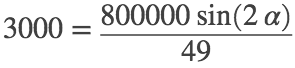ang = solve(eq2, alpha)
ang =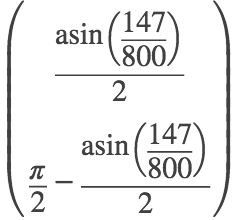0.0924 1.4784
deg = 2×1
5.2900 84.7100
% The latter is preferable unless shooting over a hill.

#### 7. [879/36]

• (a) Equation 7 on page 874 gives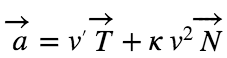, where v is speed and κ is curvature. Since the particle's path is a straight line,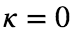, whence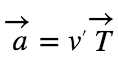and the acceleration and velocity (unit tangent) vectors are parallel.
• (b) From the same equation, if the particle moves with constant speed, then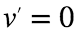, whence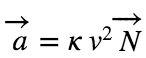and the acceleration and unit normal vectors are parallel. Thus the acceleration and velocity (unit tangent) vectors are perpendicular.

#### 8. [879/38]

Tangential and normal components of acceleration are computed.
syms t
r = [2*t^2 2/3*t^3 - 2*t 0]
r =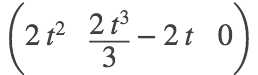v = diff(r,t), a = diff(v,t)
v =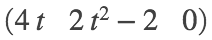a =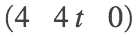aT = simplify(dot(v,a) / norm(v))
aT =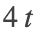aN = simplify(norm(cross(v,a)) / norm(v))
aN = 4

#### 9. [879/40]

Tangential and normal components of acceleration are computed.
syms t
r = [t 2*exp(t) exp(2*t)]
r =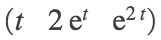v = diff(r,t), a = diff(v,t)
v =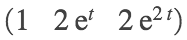a =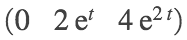aT = simplify(dot(v,a) / norm(v))
aT =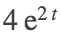aN = simplify(norm(cross(v,a)) / norm(v))
aN =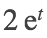#### 10. [879/42]

Tangential and normal components of acceleration are computed.
syms t positive
r = [1/t 1/t^2 1/t^3]
r =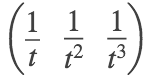v = diff(r,t), a = diff(v,t)
v =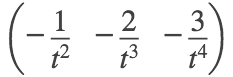a =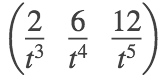aT(t) = simplify(dot(v,a) / norm(v))
aT(t) =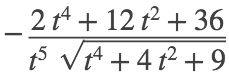aN(t) = simplify(norm(cross(v,a)) / norm(v))
aN(t) =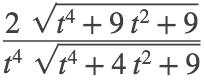aT1 = simplify(aT(1)), aN1 = simplify(aN(1))
aT1 =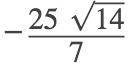aN1 =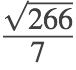chk1 = simplify(aT1 == sym(-50/sqrt(14))) % formats
chk1 = TRUE
chk2 = simplify(aN1 == sym(sqrt(38/7))) % alternative
chk2 = TRUE